# Module 2: BASIC PROGRAMMING FUNDAMENTALS

## Learning Objectives

After reading this lesson, you should be able to:

1. define a mathematical variable,

2. define a programming variable,

3. determine legal and illegal variable names,

4. know the benefits of good practices for variable naming,

5. have guidelines for naming variables.

## What is a mathematical variable?

A mathematical variable is a number that we do not know yet and may have to solve for. For example, in the simple algebraic equation $$2 + x = 3$$, the mathematical variable is $$\text{x}$$. A mathematical variable can also essentially be a placeholder for substituting a variety/range of numbers. For example, we can substitute any range of numbers into x in the function $$f(x)=x+5$$ to find the value of a function, $$f(x)$$. In a general sense, a variable varies its value. The value of a variable may be arbitrary, not specified, or even unknown.

## What is a programming variable?

In computer programming, such as MATLAB, a programming variable connects the name of a variable and a specific storage location in the computer memory. For example, the variable x references/points to its allocated storage, which contains some information about the variable; e.g., the value you assigned to it. Figure 1 shows a simple graphical illustration of this concept.

Although a variable in computer programming can be used as a mathematical variable, it can also be used for many more applications such as substitution, information storage, iteration, value comparison, and much more. Do not worry if you are not sure how to do any of these in MATLAB yet; subsequent lessons will explain how to use variables for all of these.

## How can I give my results a variable name of their own?

You have seen variables used in previous lessons, but you did not know the specifics about the concept or how to name them in MATLAB. To make sense of the information that you are receiving and inputting into MATLAB, you can assign names to the input, intermediate, and output variables. MATLAB allows you to name variables simply by typing the desired name followed by an equal to sign and then the operation. For instance, if you are using MATLAB to find the area of a square, you may type, areaSq, then press the “equal to” key followed by the operation of length times width. This will return the value of the area. You can also recall or use this expression in a later operation simply by typing in the variable name wherever it is needed. This is shown in Example 1.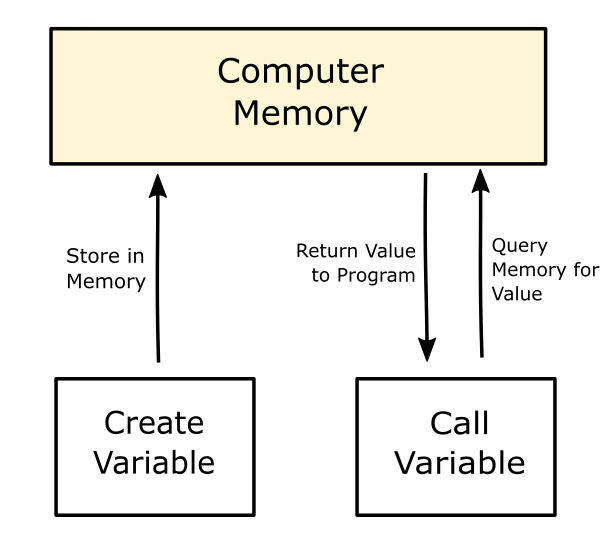Figure 1: A simple visual representation of how variables are saved and called.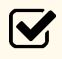Important Note: When naming variables, you cannot start the variable name with a number or use a space in the name. For example, 1cat and cat 1 are illegal variable names. Also, cos is an illegal variable name because it is used as a MATLAB function to calculate the cosine of an angle.

We will learn the MATLAB functions and commands later (throughout the rest of the book). MATLAB is case sensitive, and hence some programmers only use lower case script for variable names.

### Example 1

Show examples of storing values in variables in MATLAB.

Solution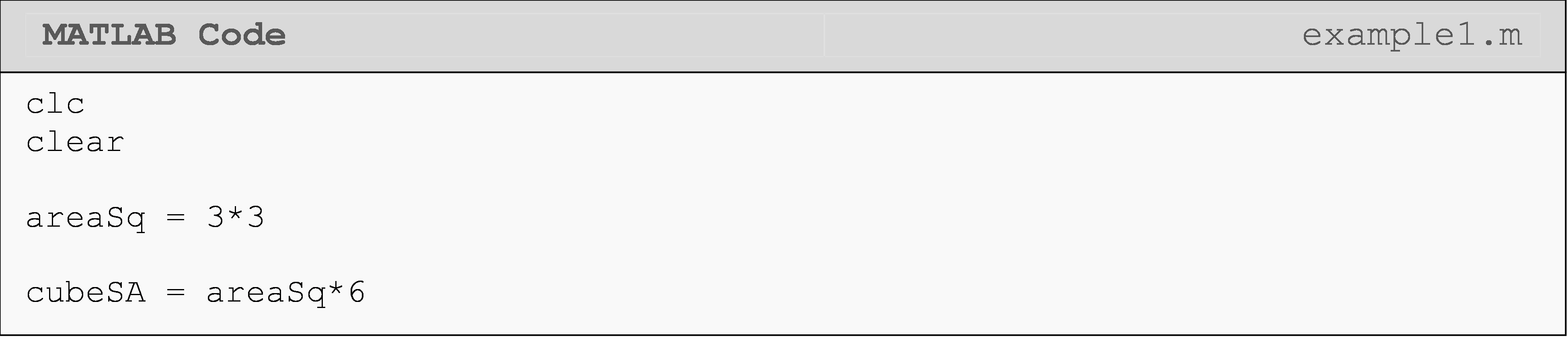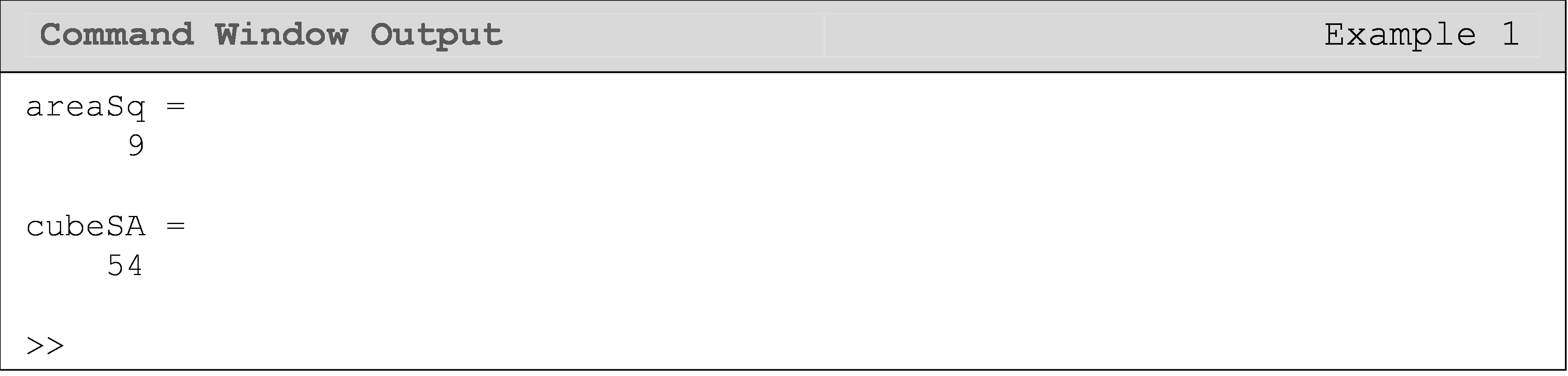Notice that in Example 1, the surface area of a cube, cubeSA, was found by using the predefined name areaSq, instead of physically typing the required dimensions to find the surface area. This is an example of recalling a previously named expression to make the current calculation easier and more readable.

## What are some possible problems with naming an expression?

You have to be cautious when naming your expressions. Follow these rules for naming.

1. Do not begin a variable name with a number.

2. Do not put a space anywhere in the variable name.

3. Do not name a variable as a predefined MATLAB command or function name.

For example,

1. 1cat is an illegal variable name, as it starts with a number.

2. cat 1 is an illegal variable name as it has a space between characters.

3. cos is an illegal variable name as it is a predefined MATLAB function that calculates the cosine of an angle.

MATLAB reads inputs from the top to the bottom and from the left to the right of the page, similar to the way you might read a book. If you are using the same variable name multiple times, MATLAB will always use the last assigned value or expression to that variable name in its calculations. Example 2 below shows an example of replacing expressions.

### Example 2

Examples of replacing an expression using the same name.

Solution

Note in the solution given below that although both SA and a are assigned values twice, the numeric values associated with those names are different. The second value of a replaces the first value and MATLAB uses this new value to calculate the next expression for SA. Both variables have been reassigned new numeric values or expressions.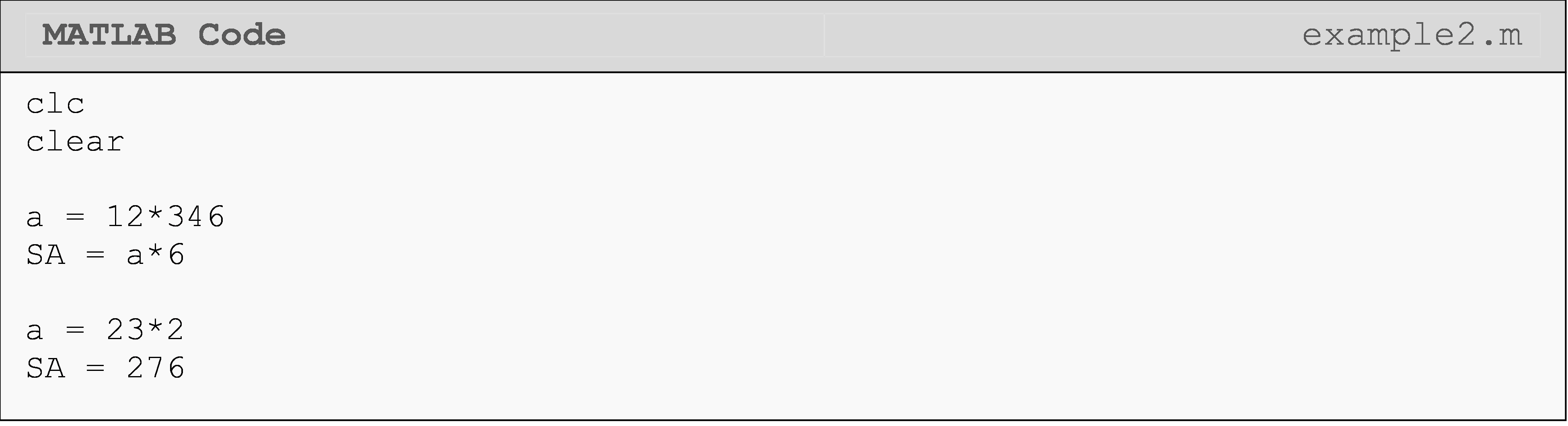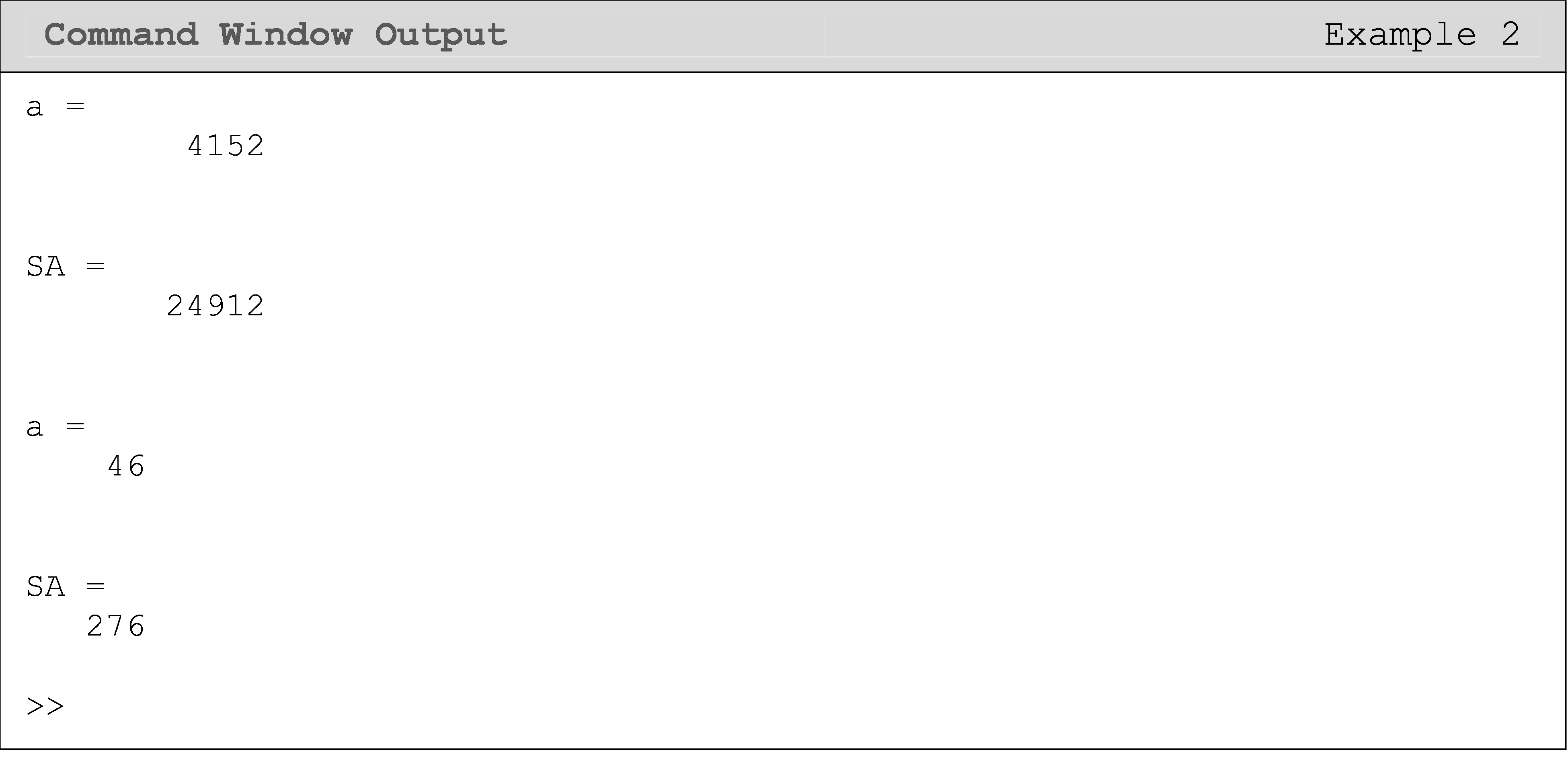## Are there benefits to good practices for variable naming?

Given that you stay within naming rules, you are free to use any variable name you wish. However, just because you can choose any variable name does not necessarily mean you should. Good variable names are essential to writing efficient and understandable code. Choosing your variable names wisely can have the following benefits:

1. Readability and clarity - It is easier to follow and understand programming code when proper variable naming techniques are followed.

2. Debugging - For more lengthy programming scripts, debugging (or troubleshooting) of code becomes more efficient and manageable.

3. Collaboration  - If you are working with someone on a piece of code, or if someone needs to read and understand your code, it is important to name your variables in a clear way.  That someone could also be you trying to figure out or reuse your code five years from now.

## Are there some guidelines for variable naming that I can follow?

This section contains a more explicit set of guidelines for naming your variables in MATLAB. These are strongly recommended; however, MATLAB will not give any errors if these are not followed. Failing to follow good naming conventions, though, can make looking at a simple program seem intimidating and frustrating. Also, note that different computer programming languages may have different naming conventions.

1. A variable covering a large scope (used across a wide range of the program) should have more specific and meaningful names.

• Good:   voltageDrop, pullForce, outputTemperature

• Bad:    vd, forP, To

2. A variable covering a small scope (used across a short range of the program) should have short, disposable names. This guideline generally applies to loop counters or dummy variables.

• Good:   i, j, elem

• Bad:    looponeiteration, temporaryVariable10

3. Use CamelCase with leading lower case letters. Note the use of underscores between words (e.g., box_height = 5) is also common; however, CamelCase will be used throughout this textbook.

• Good:   pressureSensorOutput, boxHeight, width

• Bad:    pressuresensoroutput, Boxheight, WiDtH

4. Avoid negating boolean (value of true or false) variable names (no double negatives). The concept of boolean variables will be covered in Module 5: Conditional Statements.

• Good:   isGood, isMax, error

• Bad:    isNotGood, isNotMax, noError

5. Do not make the variable name very long. Also, the maximum length of variable names is limited to 63 characters. You will have to use your own judgement beyond this constraint. In general, it must be long enough to be descriptive, yet short enough to be memorable and useful.

• Good:   avgPartStress, isTankLightOn

• Bad:    averageStressInPartThatIsConnectedToTheOtherPart,
isTheFirstLightOnTopOfTheTankFlashingGreen

The following example shows a short MATLAB script with badly selected variable names. For this example do not worry about the function of this specific MATLAB m-file script. We have included examples and explanations for each guideline to elucidate each point more clearly and hopefully impress them on your memory.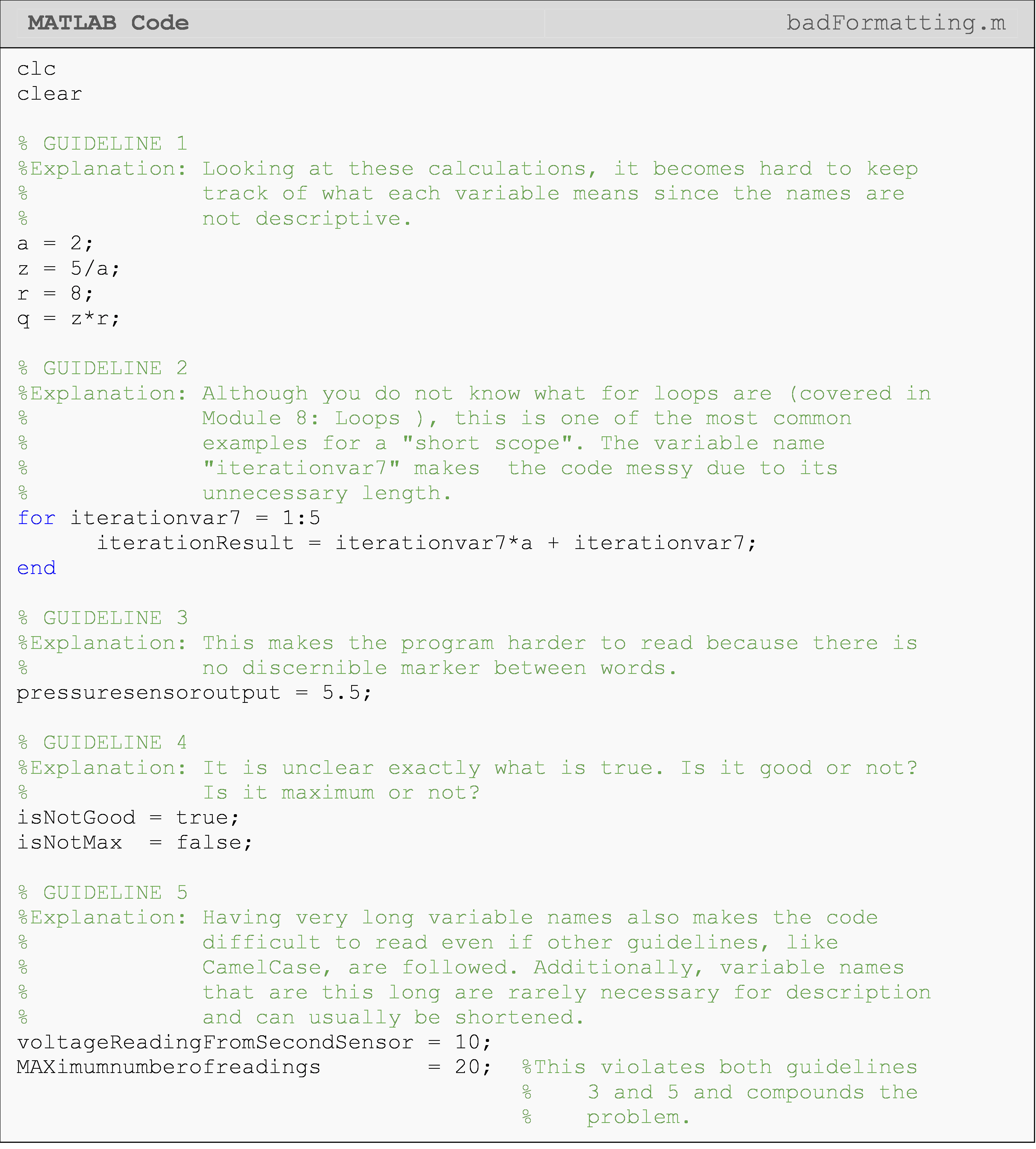In the code below, we rewrite our first MATLAB script example to put our guidelines into practice. Again, for this example do not worry about the function or meaning of this specific MATLAB m-file program. Note that the added white space and alignment further enhance readability.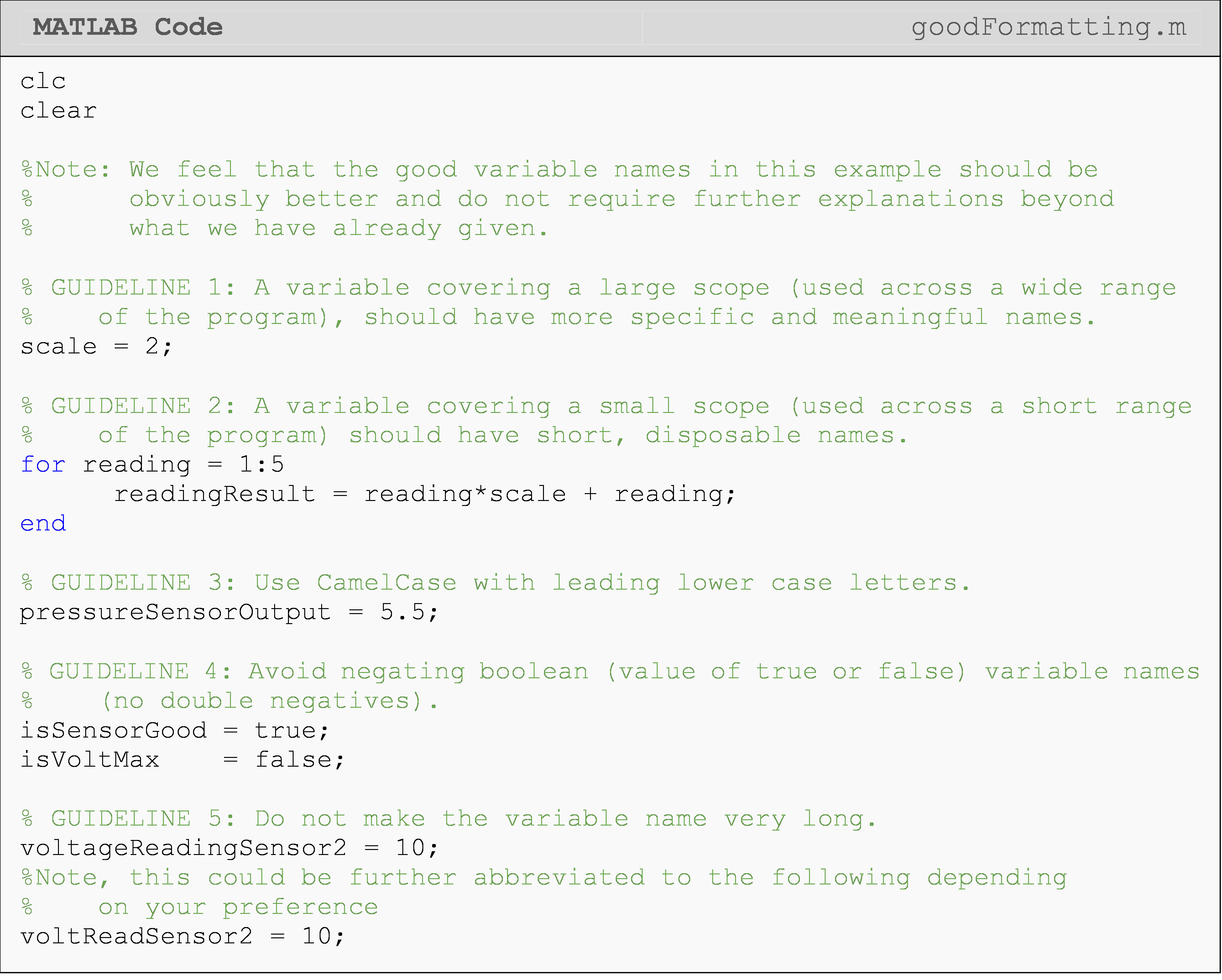## Lesson Summary of New Syntax and Programming Tools

 Task Syntax Example Usage Store a value in a programming variable validName validName = 6 validName2 = 'some text'

## Multiple Choice Quiz

(1). A correct name for a variable is

(a)  1arearec

(b)  area rec

(c)  area_rec

(d)  cos

(2). What is the mistake in the following code (m-file is saved with the name exercise_2.m)?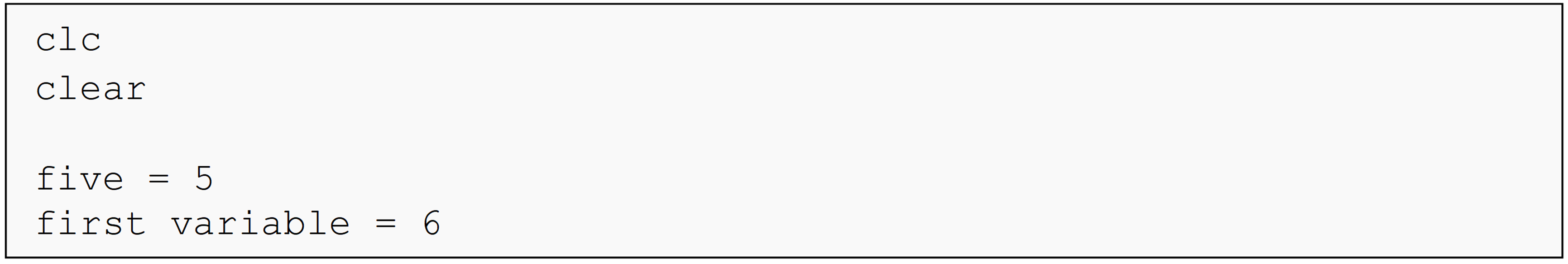(a)  The m-file name is invalid.

(b)  One of the variables is called (referenced) before it is defined (assigned a value).

(c)  One of the variable names is invalid.

(d)  None of the above

(3). An incorrect name for a variable is

(a)  cat1

(b)  cat_1

(c)  cat_cos

(d)  1cat

(4). The following variable follows the rules of camelCase.

(a)  AreaSquare

(b)  areaSquare

(c)  areasquare

(d)  Areasquare

(5). The following program is given to you. What is the value of the variable c?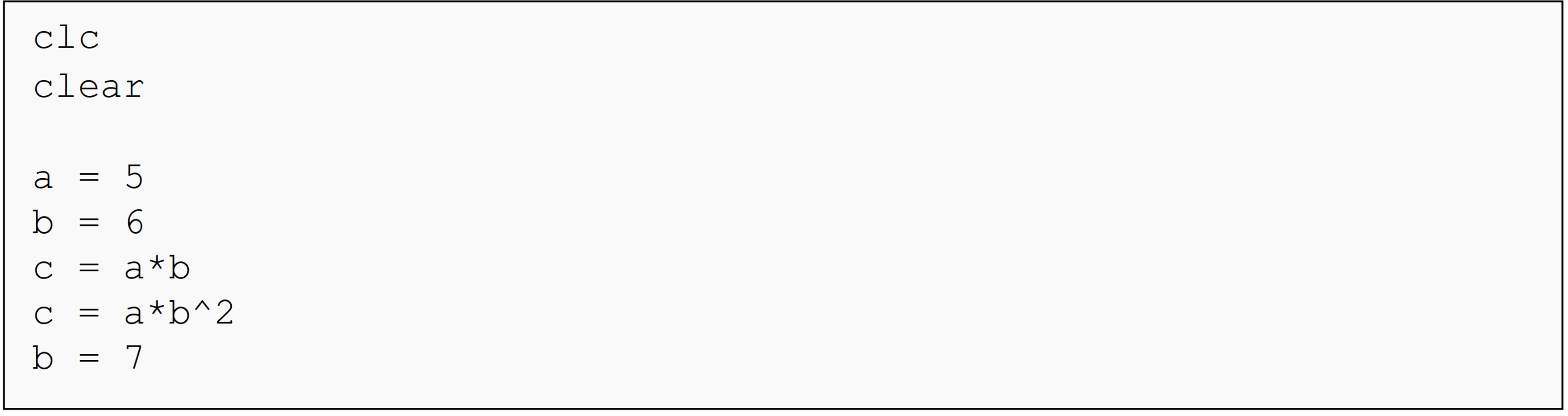(a)  30

(b)  35

(c)  180

(d)  245

## Problem Set

(1). Enumerate the benefits of good naming practices of variables.

(2). Enumerate guidelines for variable naming. Give examples of each enumeration.

(3). Enumerate illegal variable names in MATLAB. Give an example of each.

(4). Write a program with proper names and good practices that calculates the inertial force in a mass with an acceleration. The value of the mass and acceleration are inputs (you choose these values) and the inertial force is the output.

(5). Write a program with proper names and good practices that calculates the current through a resistor. The value of the resistance and voltage are known inputs (you choose these values) and the current through the resistor is the output. Remember, $$I=\displaystyle\frac{V}{R}$$.